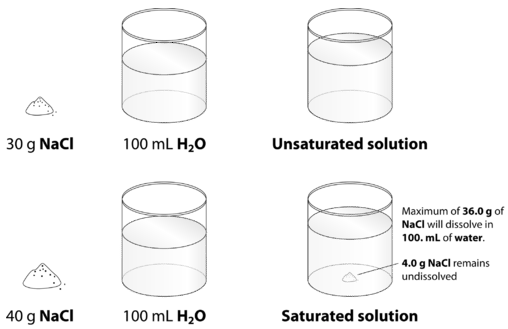# 10.1: Solubility Trends

•• Anonymous
• LibreTexts
$$\newcommand{\vecs}{\overset { \rightharpoonup} {\mathbf{#1}} }$$ $$\newcommand{\vecd}{\overset{-\!-\!\rightharpoonup}{\vphantom{a}\smash {#1}}}$$$$\newcommand{\id}{\mathrm{id}}$$ $$\newcommand{\Span}{\mathrm{span}}$$ $$\newcommand{\kernel}{\mathrm{null}\,}$$ $$\newcommand{\range}{\mathrm{range}\,}$$ $$\newcommand{\RealPart}{\mathrm{Re}}$$ $$\newcommand{\ImaginaryPart}{\mathrm{Im}}$$ $$\newcommand{\Argument}{\mathrm{Arg}}$$ $$\newcommand{\norm}{\| #1 \|}$$ $$\newcommand{\inner}{\langle #1, #2 \rangle}$$ $$\newcommand{\Span}{\mathrm{span}}$$ $$\newcommand{\id}{\mathrm{id}}$$ $$\newcommand{\Span}{\mathrm{span}}$$ $$\newcommand{\kernel}{\mathrm{null}\,}$$ $$\newcommand{\range}{\mathrm{range}\,}$$ $$\newcommand{\RealPart}{\mathrm{Re}}$$ $$\newcommand{\ImaginaryPart}{\mathrm{Im}}$$ $$\newcommand{\Argument}{\mathrm{Arg}}$$ $$\newcommand{\norm}{\| #1 \|}$$ $$\newcommand{\inner}{\langle #1, #2 \rangle}$$ $$\newcommand{\Span}{\mathrm{span}}$$$$\newcommand{\AA}{\unicode[.8,0]{x212B}}$$

##### Learning Objectives
• Define solubility and saturation.

We have learned that solutions can be formed in a variety of combinations using solids, liquids, and gases. We also know that solutions have the same composition throughout, and that the ratio of components can be varied up to a point to maintain the homogeneous nature of the solution. In this section, we will discuss how that restriction on components is defined, and how changing external conditions might change the range of possible compositions for a solution.

## Solubility and Saturation

Table salt $$\left( \ce{NaCl} \right)$$ readily dissolves in water. In most cases, only a certain maximum amount of solute can be dissolved in a given amount of solvent. This maximum amount is specified as the solubility of the solute. It is usually expressed in terms of the amount of solute that can dissolve in 100 g of the solvent at a given temperature. Table $$\PageIndex{1}$$ lists the solubilities of some simple ionic compounds. These solubilities vary widely. NaCl can dissolve up to 31.6 g per 100 g of H2O, while AgCl can dissolve only 0.00019 g per 100 g of H2O.

Table $$\PageIndex{1}$$: Solubilities of Some Ionic Compounds
Solute Solubility (g per 100 g of H2O at 25°C)
AgCl 0.00019
CaCO3 0.0006
KBr 70.7
NaCl 36.1
NaNO3 94.6

When the maximum amount of solute has been dissolved in a given amount of solvent, we say that the solution is saturated with solute. When less than the maximum amount of solute is dissolved in a given amount of solute, the solution is unsaturated. These terms are also qualitative terms because each solute has its own solubility. A solution of 0.00019 g of AgCl per 100 g of H2O may be saturated, but with so little solute dissolved, it is also rather dilute. A solution of 36.1 g of NaCl in 100 g of H2O is also saturated, but rather concentrated. In some circumstances, it is possible to dissolve more than the maximum amount of a solute in a solution. Usually, this happens by heating the solvent, dissolving more solute than would normally dissolve at regular temperatures, and letting the solution cool down slowly and carefully. Such solutions are called supersaturated solutions and are not stable; given an opportunity (such as dropping a crystal of solute in the solution), the excess solute will precipitate from the solution.The figure below illustrates the above process and shows the distinction between unsaturated and saturated.Figure $$\PageIndex{1}$$: When $$30.0 \: \text{g}$$ of $$\ce{NaCl}$$ is added to $$100 \: \text{mL}$$, it all dissolves, forming an unsaturated solution. When $$40.0 \: \text{g}$$ is added, $$36.0 \: \text{g}$$ dissolves and $$4.0 \: \text{g}$$ remains undissolved, forming a saturated solution.

How can you tell if a solution is saturated or unsaturated? If more solute is added and it does not dissolve, then the original solution was saturated. If the added solute dissolves, then the original solution was unsaturated. A solution that has been allowed to reach equilibrium, but which has extra undissolved solute at the bottom of the container, must be saturated. In the subsections that follow, we will discuss how some external properties might affect the solubility of a solution.

## Summary

• Solubility is the specific amount of solute that can dissolve in a given amount of solvent.
• Saturated and unsaturated solutions are defined.

## Vocabulary

• Miscible - Liquids that have the ability to dissolve in each other.
• Immiscible - Liquids that do not have the ability to dissolve in each other.

This page titled 10.1: Solubility Trends is shared under a mixed license and was authored, remixed, and/or curated by Anonymous.